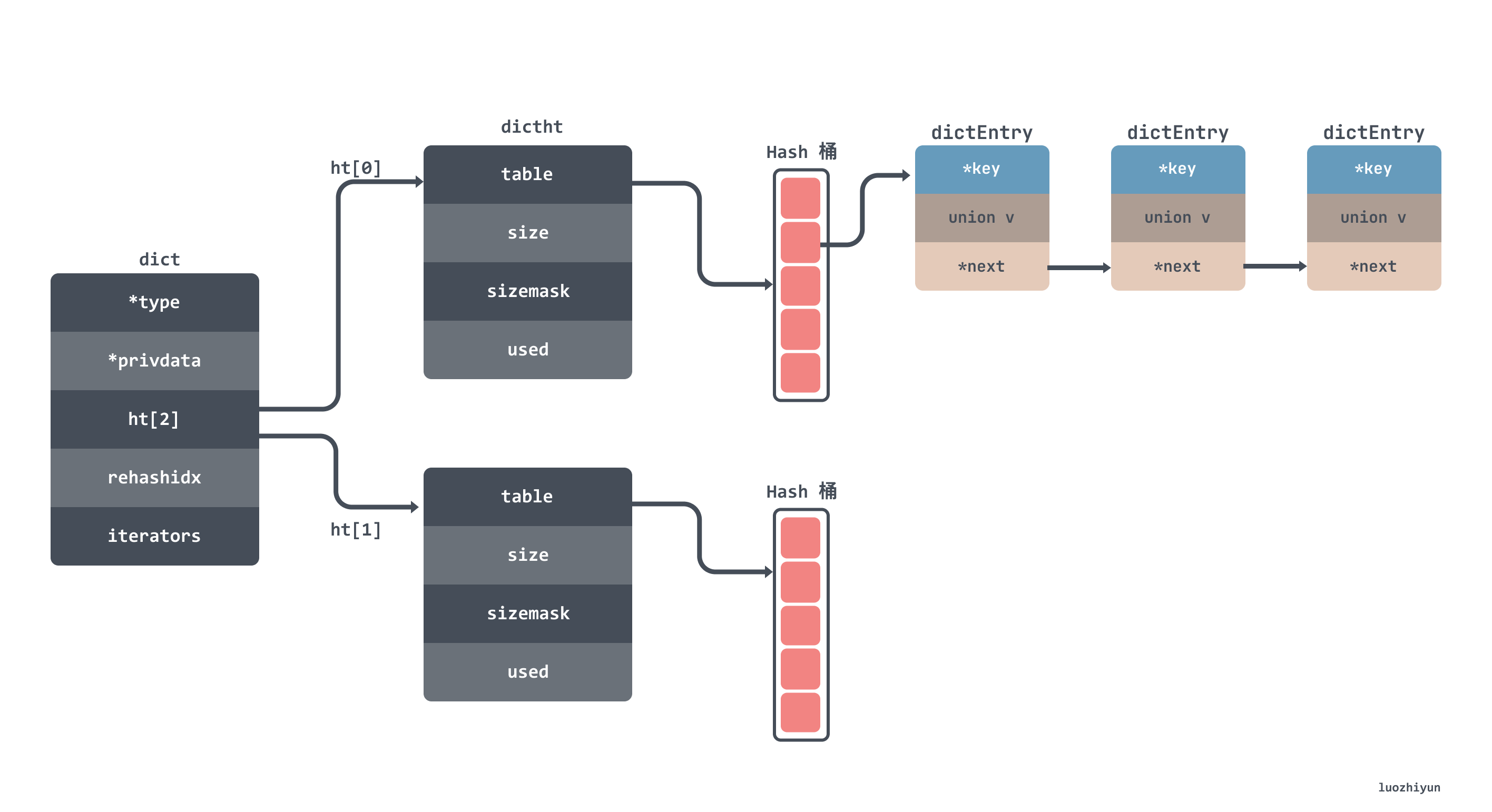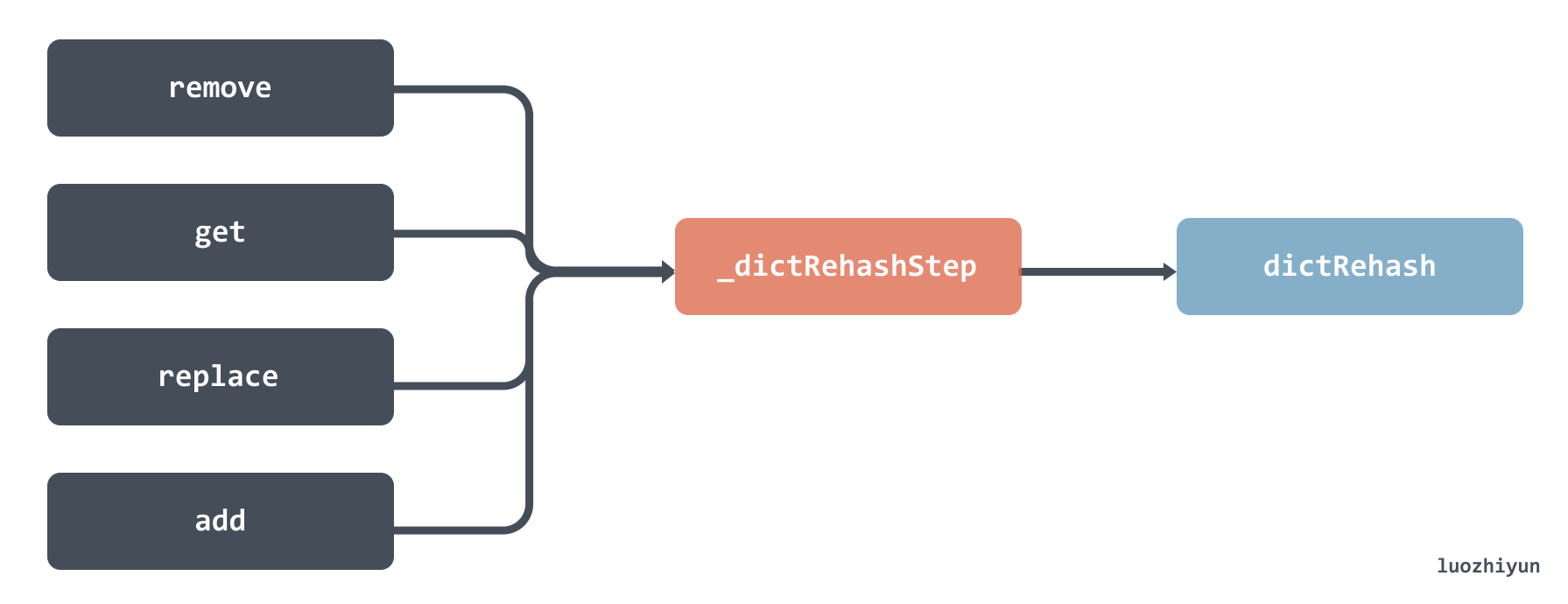# 透过Redis源码探究Hash表的实现

## 概述

1. 有没有并发操作；
2. Hash冲突如何解决；
3. 以什么样的方式扩容。Redis 在结构体中设置两个表 `ht``ht`，如果当前 `ht`的容量是 0 ，那么第一次会直接给4个容量；如果不是 0 ，那么容量会直接翻倍，然后将新内存放入到`ht`中返回，并设置标记0表示在扩容中。

## 设计与实现

Redis 的 hash 实现主要在 dict.h 和 dict.c 这两个文件中。

hash 表的数据结构大致如下所示，我就不贴出结构体的代码了，字段都标注在图上了：### 何时触发扩缩容？

#### 扩容

This function adds the entry but instead of setting a value returns the dictEntry structure to the user, that will make sure to fill the value field as he wishes.

dicAddRaw 函数会调用到 _dictKeyIndex 函数，这个函数会调用 _dictExpandIfNeeded 判断是否需要扩容。

`````` ┌─────────────┐     ┌─────────┐      ┌─────────────┐      ┌─────────────────────┐
└─────────────┘     └─────────┘      └─────────────┘      └─────────────────────┘``````

`_dictExpandIfNeeded` 函数判断了大致有三种情况会进行扩容：

1. 如果 hash 表的size为0，那么创建一个容量为4的hash表；
2. 服务器目前没有在执行 rdb 或者 aof 操作， 并且哈希表的负载因子大于等于 `1`
3. 服务器目前正在执行 rdb 或者 aof 操作， 并且哈希表的负载因子大于等于 `5`

``````// load ratio = the number of elements / the buckets

``load_ratio = 4 / 4 = 1``

``load_ratio = 256 / 512 = 0.5``

as we use copy-on-write and don’t want to move too much memory around when there is a child performing saving operations.

``````static int _dictExpandIfNeeded(dict *d)
{
// 正在扩容中
if (dictIsRehashing(d)) return DICT_OK;
// 如果 hash 表的size为0，那么创建一个容量为4的hash表
if (d->ht.size == 0) return dictExpand(d, DICT_HT_INITIAL_SIZE);

// hash表中元素的个数已经大于hash表桶的数量
if (d->ht.used >= d->ht.size &&
//dict_can_resize 表示是否可以扩容
(dict_can_resize ||
// hash表中元素的个数已经除以hash表桶的数量是否大于5
d->ht.used/d->ht.size > dict_force_resize_ratio))
{
return dictExpand(d, d->ht.used*2); // 容量扩大两倍
}
return DICT_OK;
}``````

``````int dictExpand(dict *d, unsigned long size)
{
//正在扩容，直接返回
if (dictIsRehashing(d) || d->ht.used > size)
return DICT_ERR;

dictht n;
// _dictNextPower会返回 size 最接近的2的指数值
// 也就是size是10，那么返回 16，size是20，那么返回32
unsigned long realsize = _dictNextPower(size);

// 校验扩容之后的值是否和当前一样
if (realsize == d->ht.size) return DICT_ERR;
// 初始化 dictht 成员变量
n.size = realsize;
n.table = zcalloc(realsize*sizeof(dictEntry*)); // 申请空间是 size * Entry的大小
n.used = 0;

//校验hash 表是否初始化过，没有初始化不应该进行rehash
if (d->ht.table == NULL) {
d->ht = n;
return DICT_OK;
}
//将新的hash表赋值给 ht
d->ht = n;
d->rehashidx = 0;
return DICT_OK;
}``````

#### 缩容

Redis 的定时器会每100ms调用一次 databasesCron 函数，它会调用到 dictResize 函数进行缩容：

`````` ┌─────────────┐   ┌──────────────────┐   ┌──────────┐   ┌──────────┐
│databasesCron├──►│tryResizeHashTable├──►│dictResize├──►│dictExpand│
└─────────────┘   └──────────────────┘   └──────────┘   └──────────┘``````

``````int dictResize(dict *d)
{
int minimal;

if (!dict_can_resize || dictIsRehashing(d)) return DICT_ERR;
minimal = d->ht.used; // 将bucket 缩小为和被键值对同样大小
if (minimal < DICT_HT_INITIAL_SIZE)
minimal = DICT_HT_INITIAL_SIZE;
return dictExpand(d, minimal);
}``````

### 数据迁移如何进行？

#### 增删改查哈希表时触发1. 校验当前迁移的bucket数量是否已达上线，并且ht是否还有元素；
2. 判断当前的迁移的bucket槽位是否为空，最大访问的空槽数量不能超过 n*10，n是本次迁移bucket数量；
3. 获取到非空槽位里面 entry 链表进行循环迁移；
1. 首先获取ht新槽位的index；
2. 一个个节点放置到新bucket的头部；
3. 直到全部迁移完毕；
4. 迁移完了将旧的hash表ht对应的bucket置空；
5. 检查如果已经rehash完了，那么需要free掉内存占用，并将ht赋值给ht；

``````int dictRehash(dict *d, int n) {
// 最大的空bucket访问次数
int empty_visits = n*10; /* Max number of empty buckets to visit. */
if (!dictIsRehashing(d)) return 0;
// 校验当前迁移的bucket数量是否已达上线，并且ht是否还有元素；
while(n-- && d->ht.used != 0) {
dictEntry *de, *nextde;

assert(d->ht.size > (unsigned long)d->rehashidx);
// 判断当前的迁移的bucket槽位是否为空
while(d->ht.table[d->rehashidx] == NULL) {
d->rehashidx++;
if (--empty_visits == 0) return 1;
}
// 获取到槽位里面 entry 链表
de = d->ht.table[d->rehashidx];
// 从老的bucket迁移数据到新的bucket中
while(de) {
uint64_t h;
nextde = de->next;
// hash之后获取新hash表的bucket槽位
h = dictHashKey(d, de->key) & d->ht.sizemask;
// 一个个节点放置到新bucket的头部
de->next = d->ht.table[h];
d->ht.table[h] = de;
d->ht.used--;
d->ht.used++;
de = nextde;
}
// 迁移完了将旧的hash表对应的bucket置空
d->ht.table[d->rehashidx] = NULL;
d->rehashidx++;
}
// 如果已经rehash完了，那么需要free掉内存占用，并将ht赋值给ht
if (d->ht.used == 0) {
zfree(d->ht.table);
d->ht = d->ht;
_dictReset(&d->ht);
d->rehashidx = -1;
return 0;// 返回0表示迁移已完成
}
return 1; // 返回1表示迁移未完成
}``````

#### 定时触发

``````  ┌─────────────┐   ┌───────────────────┐   ┌──────────────────────┐   ┌──────────┐
│databasesCron├──►│incrementallyRehash├──►│dictRehashMilliseconds├──►│dictRehash│
└─────────────┘   └───────────────────┘   └──────────────────────┘   └──────────┘``````

dictRehashMilliseconds 函数传入的 ms 参数表示可以运行多长时间，默认传入的是1，也就是运行1ms就会退出这个函数：

``````int dictRehashMilliseconds(dict *d, int ms) {
long long start = timeInMilliseconds();
int rehashes = 0;
// 每次会迁移 100 个 bucket
while(dictRehash(d,100)) {
rehashes += 100;
if (timeInMilliseconds()-start > ms) break;
}
return rehashes;
}``````

## Reference

https://tech.meituan.com/2018/07/27/redis-rehash-practice-optimization.html

http://redisbook.com/preview/dict/rehashing.html